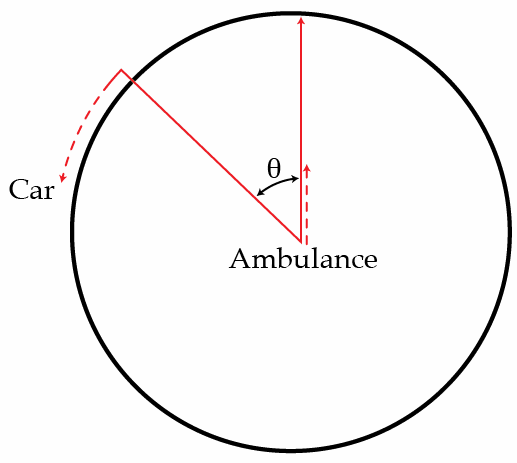# Avoiding in a circle

An ambulance car is going with a speed of $60~\mbox{km/h}$, while a car is trying to go around it in a circle with a speed $50~\mbox{km/h}$. If the sound that ambulance car emits has a frequency of $1~\mbox{kHz}$, which frequency does the driver of the car hear in Hz when the ambulance car is in the center of the circle it makes, and the car makes an angle $\theta = 30^\circ$ with the direction of the car?Details and assumptions

• Speed of sound in the air is $c = 1235~\mbox{km/h}$.
×